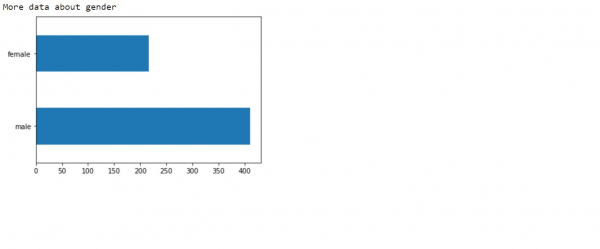# How Tensorflow be used for the gender column, like feature column helping in prediction?

TensorflowServer Side ProgrammingProgramming

The ‘gender’ column will be used in the prediction as well, hence it is important to understand more about it. This can be done by visualizing the ‘age’ column as a horizontal bar plot.

We will use the Keras Sequential API, which is helpful in building a sequential model that is used to work with a plain stack of layers, where every layer has exactly one input tensor and one output tensor.

A neural network that contains at least one layer is known as a convolutional layer. We can use the Convolutional Neural Network to build learning model.

We are using the Google Colaboratory to run the below code. Google Colab or Colaboratory helps run Python code over the browser and requires zero configuration and free access to GPUs (Graphical Processing Units). Colaboratory has been built on top of Jupyter Notebook.

An Estimator is TensorFlow's high-level representation of a complete model. It is designed for easy scaling and asynchronous training.  We will train a logistic regression model using the tf.estimator API. The model is used as a baseline for other algorithms. We use the titanic dataset with the goal of predicting passenger survival, given characteristics such as gender, age, class, etc.

## Example

print("More data about gender")
dftrain.sex.value_counts().plot(kind='barh')
plt.show()

## Output## Explanation

• The gender column will be useful in the prediction process further.
• Hence it is visualized on the console.